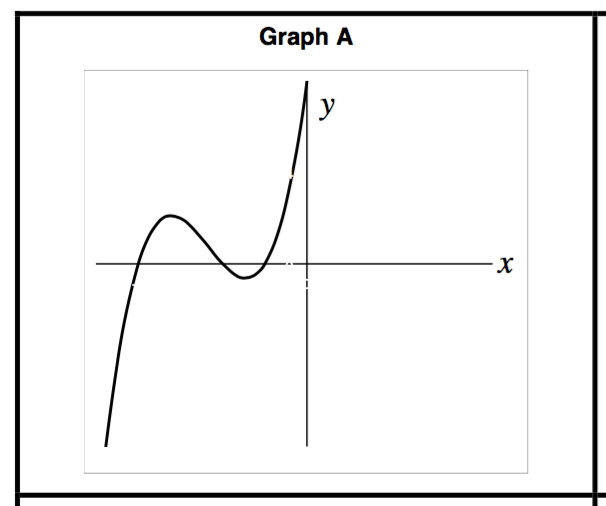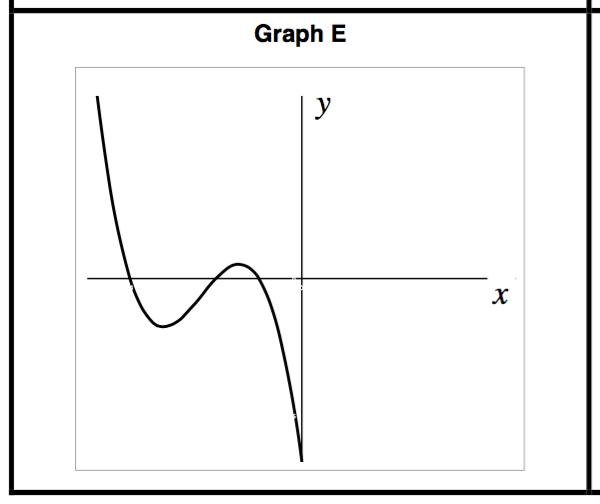# Polynomials

## Objective

Match and compare equations and graphs of polynomials, and identify transformations.

## Common Core Standards

### Core Standards

?

• F.BF.B.3 — Identify the effect on the graph of replacing f(x) by f(x) + k, k f(x), f(kx), and f(x + k) for specific values of k (both positive and negative); find the value of k given the graphs. Experiment with cases and illustrate an explanation of the effects on the graph using technology. Include recognizing even and odd functions from their graphs and algebraic expressions for them.

• F.IF.B.4 — For a function that models a relationship between two quantities, interpret key features of graphs and tables in terms of the quantities, and sketch graphs showing key features given a verbal description of the relationship. Key features include: intercepts; intervals where the function is increasing, decreasing, positive, or negative; relative maximums and minimums; symmetries; end behavior; and periodicity. Modeling is best interpreted not as a collection of isolated topics but in relation to other standards. Making mathematical models is a Standard for Mathematical Practice, and specific modeling standards appear throughout the high school standards indicated by a star symbol (★). The star symbol sometimes appears on the heading for a group of standards; in that case, it should be understood to apply to all standards in that group.

• F.IF.C.7.C — Graph polynomial functions, identifying zeros when suitable factorizations are available, and showing end behavior.

?

• F.IF.C.9

## Criteria for Success

?

1. Use the features of a polynomial function of end behavior, roots, and leading coefficient to match polynomial functions with their equations.
2. Identify transformations of functions based on their roots.
3. Use transformations of functions to make inferences about the end behavior of functions.
4. Describe whether a set of described features is enough to give one possible equation of the function.

## Anchor Problems

?

### Problem 1

Below are the sketches of two different functions. Which one matches with the equation shown below?${y=(x+1)(x+2)(x+4)}$

#### References

MARS Formative Assessment Lessons for High School Representing Polynomials Graphically

Representing Polynomials Graphically from the Classroom Challenges by the MARS Shell Center team at the University of Nottingham is made available by the Mathematics Assessment Project under the CC BY-NC-ND 3.0 license. Copyright © 2007-2015 Mathematics Assessment Resource Service, University of Nottingham. Accessed Sept. 25, 2017, 12:17 p.m..

### Problem 2

Is the following statement always, sometimes, or never true?

"If ${ f(x)}$ is a cubic function and ${ f(1)=0}$, ${f(3)=0}$, and ${ f(4)=0}$, then ${ f(5)}$ could be any number but zero."

#### References

MARS Formative Assessment Lessons for High School Representing Polynomials GraphicallyTrue false statements, card C2

Representing Polynomials Graphically from the Classroom Challenges by the MARS Shell Center team at the University of Nottingham is made available by the Mathematics Assessment Project under the CC BY-NC-ND 3.0 license. Copyright © 2007-2015 Mathematics Assessment Resource Service, University of Nottingham. Accessed Sept. 25, 2017, 12:17 p.m..

## Problem Set

?

The following resources include problems and activities aligned to the objective of the lesson that can be used to create your own problem set.

The function ${y=(x-2)(x+3)^2}$ is transformed to:
1.  ${y=(x-2)(x+3)^2}+4$ . Describe in words how the function has been transformed
2.  ${y=-(x-2)(x+3)^2}$. Describe in words how the function has been transformed.
3.  ${y=(x+1)(x+6)^2}$. Describe in words how the function has been transformed.